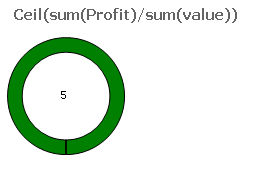# QlikView Scripting

Discussion Board for collaboration on QlikView Scripting.

Valued Contributor

## Re: Division Calculation in expression

as Per your expression  Guage chart is showing result

is 4 is it correct?

Contributor II

## Re: Division Calculation in expression

Data I have provided should give me 5.1 as result in guage

Valued Contributor

## Re: Division Calculation in expression

Hi

as per data it was showing  result is 5

Contributor II

## Re: Division Calculation in expression

yes answer is 5

Valued Contributor

## Re: Division Calculation in expression

is it okValued Contributor

## Re: Division Calculation in expression

Hi please find the attached file

Highlighted
Contributor II

## Re: Division Calculation in expression

Ceil do not give me perfect value

I want

Profitability to be calculated as

(Sum of total profit) / (Sum of total Value)

Valued Contributor

## Re: Division Calculation in expression

Hi

as per data its giving the result like

 4.69392

if u want check in text object like  :-    =sum(TOTAL  Profit)/sum( TOTAL value)

Contributor II

## Re: Division Calculation in expression

I got it

Sum(Profit)/Sum(Value) gave me the desired answer.

Thanks for the help.MVP

## Re: Division Calculation in expression

Hi Vikas, if your answer is solved please mark correct/helpful answers to close the thread.